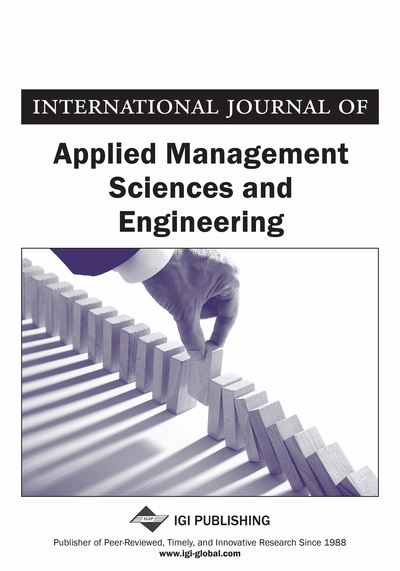# Alternative Models for Forecasting Macroeconomic Indicators in Kazakhstan

Saken Pirmakhanov (Dongbei University of Finance and Economics, Astana, Kazakhstan)
DOI: 10.4018/IJAMSE.2017010101
Available
\$37.50
No Current Special Offers

## Abstract

This paper indicates special aspects of using vector auto-regression models to forecast rates of basic macroeconomic indicators in short term. In particular, traditional vector auto-regression model, Bayesian vector auto-regression model and factor augmented vector auto-regression model are shown. For parameter estimation of these models the author uses time series of Kazakhstani macroeconomic indicators between 1996 and 2015 quarterly. In virtue of mean-root-square error prediction the conclusion of optimal model is going to be chosen.
Article Preview
Top

## 1. Introduction

For the effective monetary policy Central banks concern to improve methodology of shirt-run and long-run prediction of macroeconomic indicators. As we know, monetary policy influences to the economy after some period of time. For this reason decision makers in the monetary policy have to concern what are going to be happened with rates of macroeconomic indicators in the future. That’s why Central banks develop structural models of equilibrium which explain structural changes in the economy as well as for long-term prediction. On the other hand, it is known that for the long-run rates of macroeconomic indicators shirt-run variation is very important as eventually shirt-run variations formulate the long-run trend. Consequently, practical using of shirt-term forecasting has a special importance for the long-run prediction. The experience of Central banks shows that for the shirt-run forecasting of macroeconomic indicators it is very advisable to use econometric models based on time series analysis, in particular vector auto-regression.

This study examines three common-used in practice short-term vector auto-regression models: unrestricted vector auto-regression (VAR), Bayesian vector auto-regression (BVAR) and factor augmented vector auto-regression (FAVAR). These vector auto-regression models are very from each other. Thus, for VAR model we can use small number of time series, whereas in contrast FAVAR model can be used by large number of time series. Secondly, VAR model has no limitations, BVAR model has limits. Thirdly, FAVAR model, as distinguished from VAR and BVAR, besides time series also includes variation of principal components. The calculation of principal components usually is based on co-variation (or correlation) matrix of initial time series and, what is more, the number of calculated principal components is considerably smaller than number of time series. There are so many calculations methods of principal components in the econometric theory among which common algorithms are static algorithm (Stock & Watson, 2002) and two-dynamic algorithm from Forni, Hallin, Lippi & Reichlin (2005), and from Doz, Giannone & Reichlin (2011, 2012). In this study, the combination of static algorithm (Stock & Watson, 2002) and dynamic algorithm from Doz, Giannone & Reichlin (2011, 2012) is used.

For the estimation of above models we used the time series of Kazakhstani macroeconomics indicators from 1996 to 2014 (quarterly). Some of macroeconomic time series are put on initial statistical elaboration, in particular, some of time series had been taken to the logarithm, seasonally fitted and their first differences are taken to account.

The main objective of this study is on the basis comparison analysis of forecasting properties of each model to find an optimal one, which will be relevant as regards minimization error mean square for the prediction of macroeconomic indicators’ rates in Kazakhstan. To find an optimal model we make experiments on the basis given time series. The point of these experiments is: total number of experimental observation we divide by two and then first part of observation we use for the estimation different models of vector auto-regression, and second part of observations use to compare with obtained forecasts of each vector auto-regression model. Generating forecasts of each vector auto-regression model made by recursion scheme regression observations. And the vector auto-regression model, which minimizes indicator of mean-root-square error of the forecast, shall be taken to be optimal.

## Complete Article List

Search this Journal:
Reset
Open Access Articles
Volume 9: 2 Issues (2022): 1 Released, 1 Forthcoming
Volume 8: 2 Issues (2021)
Volume 7: 2 Issues (2020)
Volume 6: 2 Issues (2019)
Volume 5: 2 Issues (2018)
Volume 4: 2 Issues (2017)
Volume 3: 2 Issues (2016)
Volume 2: 2 Issues (2015)
Volume 1: 2 Issues (2014)
View Complete Journal Contents Listing